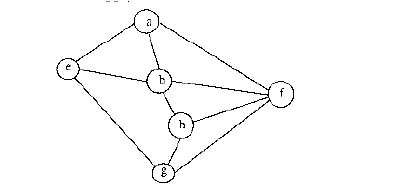# Data Structures and Algorithms | Set 6

Following questions have been asked in GATE CS exam.

1. The usual Θ(n^2) implementation of Insertion Sort to sort an array uses linear search to identify the position where an element is to be inserted into the already sorted part of the array. If, instead, we use binary search to identify the position, the worst case running time will (GATE CS 2003)
(a) remain Θ(n^2)
(b) become Θ(n(log n)^2)
(c) become Θ(n log n)
(d) become Θ(n)

If we use binary search then there will be ⌈ Log2(n!) ⌉ comparisons in the worst case, which is Θ(n log n) ( If you want to know how ⌈ Log2(n!) ⌉ can be equal to Θ(n log n)), then see this for proof). But the algorithm as a whole will still have a running time of Θ(n^2) on average because of the series of swaps required for each insertion.

2. The tightest lower bound on the number of comparisons, in the worst case, for comparison-based sorting is of the order of

a) n
b) n^2
c) nlogn
d) n(log^2)n

The number of comparisons that a comparison sort algorithm requires increases in proportion to nlog(n), where n is the number of elements to sort. This bound is asymptotically tight:

Given a list of distinct numbers (we can assume this because this is a worst-case analysis), there are n factorial permutations exactly one of which is the list in sorted order. The sort algorithm must gain enough information from the comparisons to identify the correct permutations. If the algorithm always completes after at most f(n) steps, it cannot distinguish more than 2^f(n) cases because the keys are distinct and each comparison has only two possible outcomes. Therefore,

```
2^f(n) >= n!, or equivalently f(n) > Log2(n!).
```

3. The problem 3-SAT and 2-SAT are

a) both in P
b) both NP complete
c) NP-complete and in P respectively
d) undecidable and NP-complete respectively

The Boolean satisfiability problem (SAT) is a decision problem, whose instance is a Boolean expression written using only AND, OR, NOT, variables, and parentheses. The problem is: given the expression, is there some assignment of TRUE and FALSE values to the variables that will make the entire expression true? A formula of propositional logic is said to be satisfiable if logical values can be assigned to its variables in a way that makes the formula true.

3-SAT and 2-SAT are special cases of k-satisfiability (k-SAT) or simply satisfiability (SAT), when each clause contains exactly k = 3 and k = 2 literals respectively.

2-SAT is P while 3-SAT is NP Complete. (See this for explanation)

4. Consider the following graphAmong the following sequences
I) a b e g h f
II) a b f e h g
III) a b f h g e
IV) a f g h b e
Which are depth first traversals of the above graph? (GATE CS 2003)

a) I, II and IV only
b) I and IV only
c) II, III and IV only
d) I, III and IV only